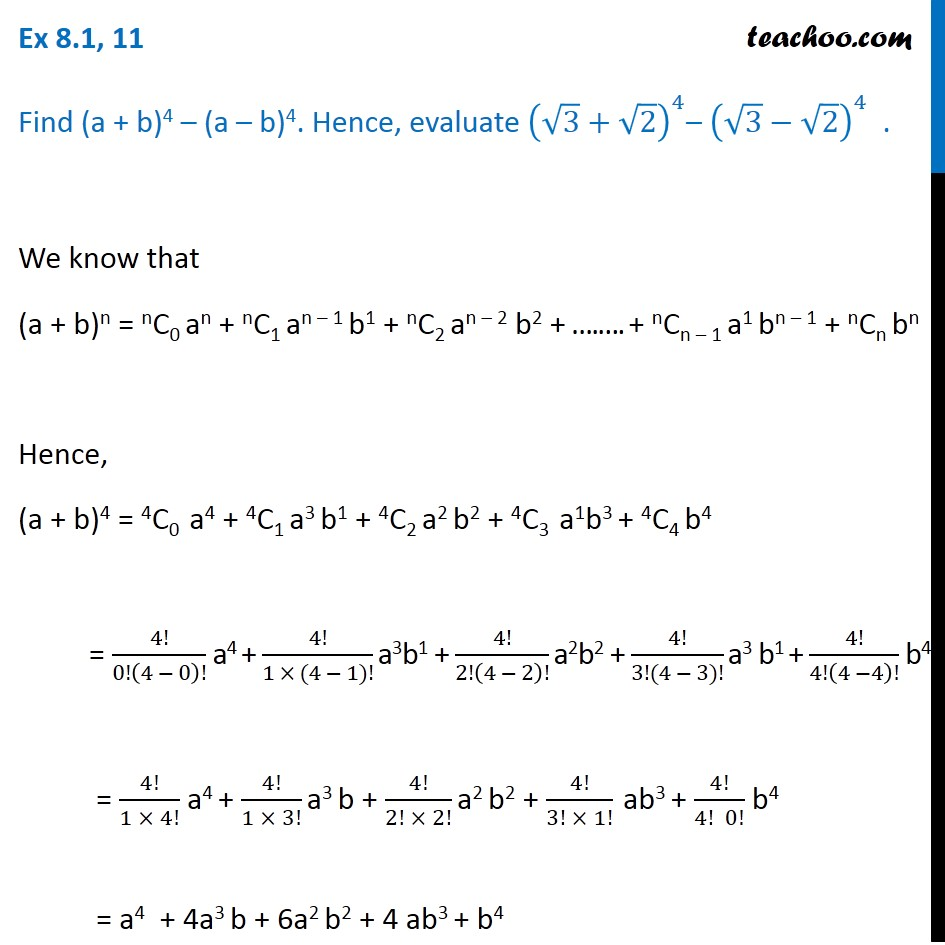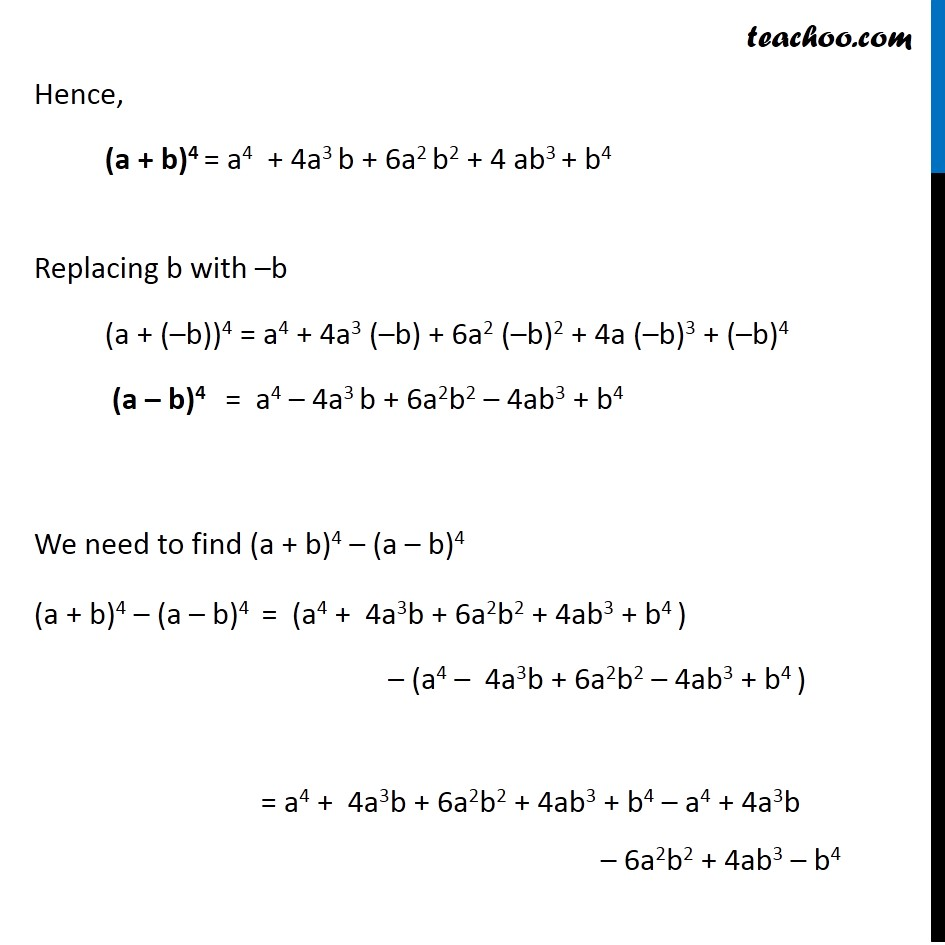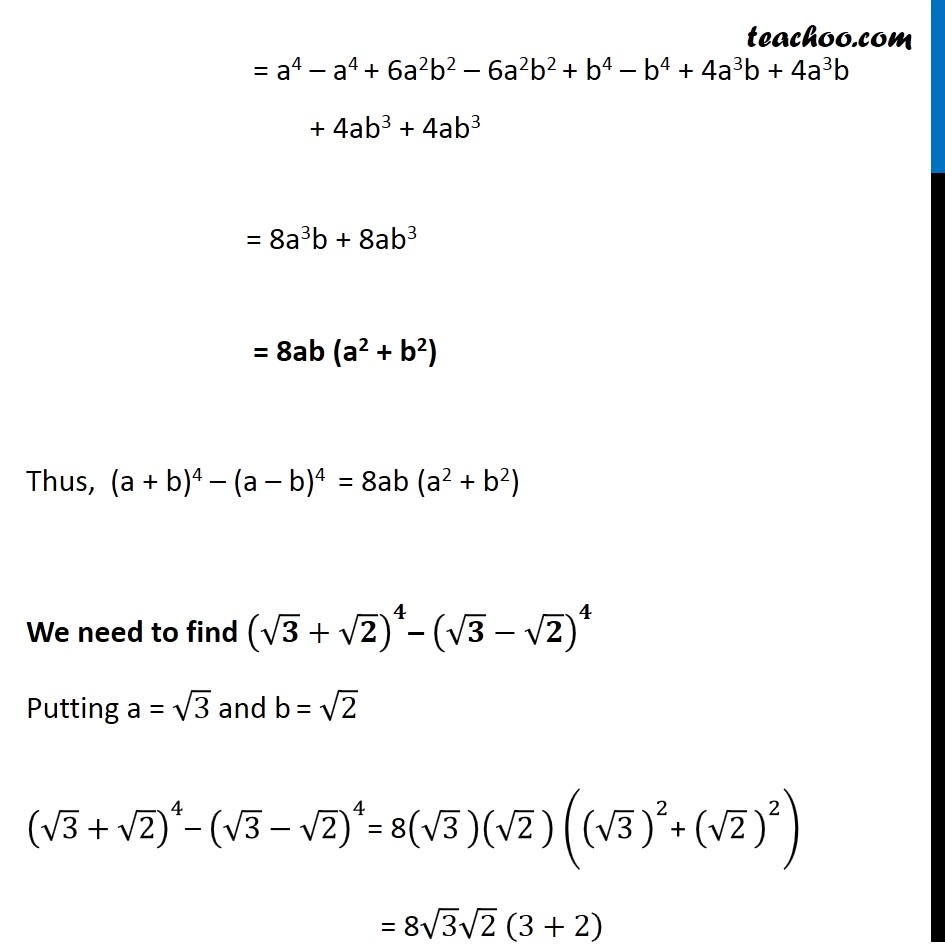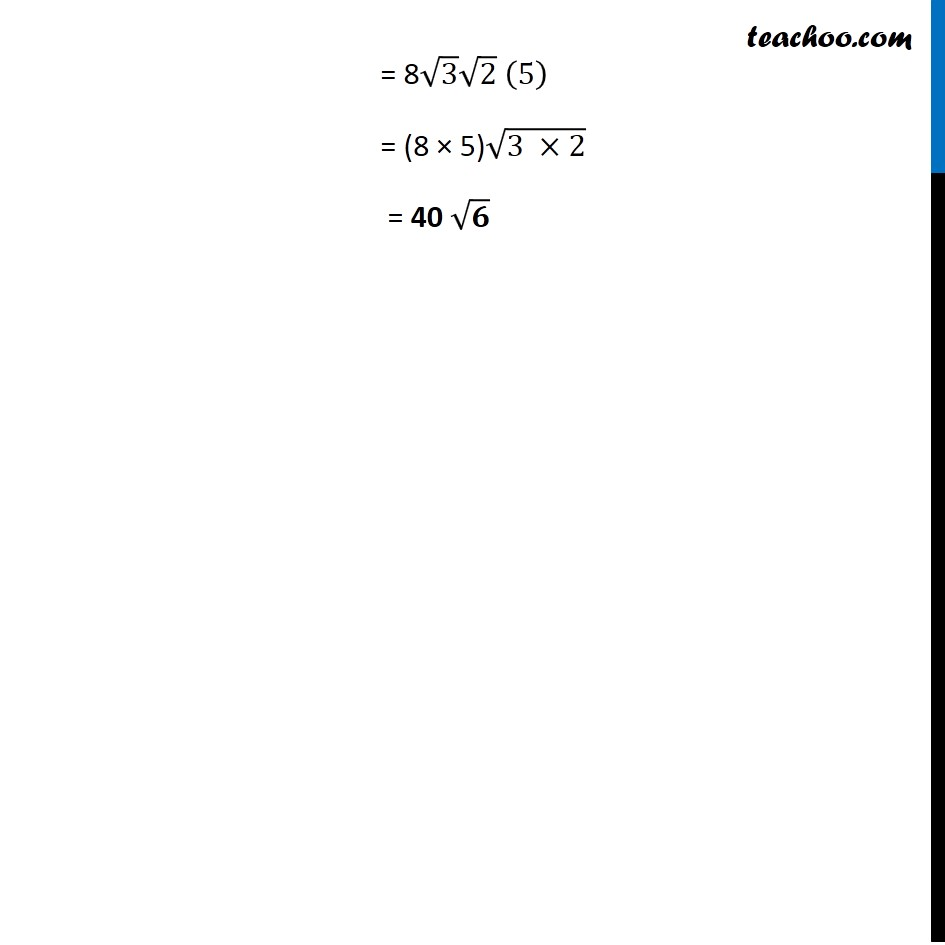Ex 7.1

Chapter 7 Class 11 Binomial Theorem
Serial order wiseLearn in your speed, with individual attention - Teachoo Maths 1-on-1 Class

### Transcript

Ex 7.1, 11 Find (a + b)4 – (a – b)4. Hence, evaluate (√3+√2)^4– (√3−√2)^4 . We know that (a + b)n = nC0 an + nC1 an – 1 b1 + nC2 an – 2 b2 + ….…. + nCn – 1 a1 bn – 1 + nCn bn Hence, (a + b)4 = 4C0 a4 + 4C1 a3 b1 + 4C2 a2 b2 + 4C3 a1b3 + 4C4 b4 = 4!/0!(4 − 0)! a4 + 4!/(1 × (4 − 1)!) a3b1 + 4!/2!(4 − 2)! a2b2 + 4!/(3!(4 − 3)!) a3 b1 + 4!/4!(4 −4)! b4 = 4!/(1 × 4!) a4 + 4!/(1 × 3!) a3 b + 4!/(2! × 2!) a2 b2 + 4!/(3! × 1!) ab3 + 4!/(4! 0!) b4 = a4 + 4a3 b + 6a2 b2 + 4 ab3 + b4 Hence, (a + b)4 = a4 + 4a3 b + 6a2 b2 + 4 ab3 + b4 Replacing b with –b (a + (–b))4 = a4 + 4a3 (–b) + 6a2 (–b)2 + 4a (–b)3 + (–b)4 (a – b)4 = a4 – 4a3 b + 6a2b2 – 4ab3 + b4 We need to find (a + b)4 – (a – b)4 (a + b)4 – (a – b)4 = (a4 + 4a3b + 6a2b2 + 4ab3 + b4 ) – (a4 – 4a3b + 6a2b2 – 4ab3 + b4 ) = a4 + 4a3b + 6a2b2 + 4ab3 + b4 – a4 + 4a3b – 6a2b2 + 4ab3 – b4 = a4 – a4 + 6a2b2 – 6a2b2 + b4 – b4 + 4a3b + 4a3b + 4ab3 + 4ab3 = 8a3b + 8ab3 = 8ab (a2 + b2) Thus, (a + b)4 – (a – b)4 = 8ab (a2 + b2) We need to find (√𝟑+√𝟐)^𝟒– (√𝟑−√𝟐)^𝟒 Putting a = √3 and b = √2 (√3+√2)^4– (√3−√2)^4= 8(√3 )(√2 ) ((√3 )^2 "+ " (√2 )^2 ) = 8√3 √2 (3+2) = 8√3 √2 (5) = (8 × 5)√(3 ×2) = 40 √𝟔